# Pressure Bulb or Stress Isobar ConceptReading time: 1 minute

## Stress Isobar or Pressure Bulb Concept

An isobar or pressure bulb is a stress contour or a line which connects all points below the ground surface at which the vertical pressure is the same. In fact, an isobar is a spatial curved surface and resembles a bulb in shape; this is because the vertical pressure at all points in a horizontal plane at equal radial distances from the load is the same. Thus, the stress isobar is also called the ‘bulb of pressure’ or simply the ‘pressure bulb’. The vertical pressure at each point on the pressure bulb is the same. Pressure at points inside the bulb are greater than that at a point on the surface of the bulb; and pressures at points outside the bulb are smaller than that value. Any number of pressure bulbs may be drawn for any applied load, since each one corresponds to an arbitrarily chosen value of stress. A system of isobars indicates the decrease in stress intensity from the inner to the outer ones and reminds one of an ‘Onion bulb’. Hence the term ‘pressure bulb’. An isobar diagram, consisting of a system of isobars appears somewhat as shown in Fig. 1 and Fig. 2.Fig.1: Pressure bulb for point loadsFig. 2: Pressure isobar for footing

## Procedure for plotting pressure bulb

The procedure for plotting an isobar is as follows: 1. Let it be required to plot an isobar for which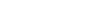per unit area (10% isobar). Stress distribution is given by formula: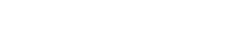where,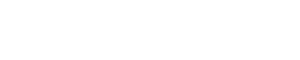Thus,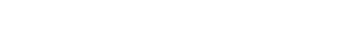2. Assuming various values for z, the corresponding KB-values are computed. 3. For the computed values of KB, the corresponding r/z-values are obtained. 4. For the assumed values of z,  r-values can be calculated. It is obvious that, for the same value of r on any side of the z-axis, or line of action of the point load, the value of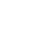is the same; hence the isobar is symmetrical with respect to this axis. So, the other half can be drawn from symmetry. When r= 0, KB= 0.4775; the isobar crosses the line of action of the load at a depth of: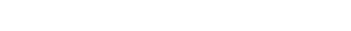The calculations are best performed in the form of a table as given below:

Table 1: Data for isobar ofper unit area.

 Depth z (units) Influence coefficients KB r/z r (units)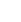0.5 0.0250 1.501 0.750 0.1Q 1.0 0.1000 0.932 0.932 0.1Q 1.5 0.2550 0.593 0.890 0.1Q 2.0 0.4000 0.271 0.542 0.1Q 2.185 0.4775 0 0 0.1Q